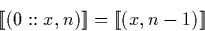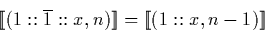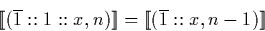Next: Dyadic (mantissa, exponent) normalisation' Up: Normalisation' Previous: Normalisation'

### Signed binary (mantissa, exponent) normalisation'

Observe that using the signed binary (mantissa, exponent) representation:Therefore if a number in (mantissa, exponent) representation is observed which starts with a zero () digit, it is possible to remove the zero and represent the original real by decrementing the exponent.

The `normalisation' function examines the head of the mantissa stream and if the first digit is a zero then the real is re-represented as shown. The function then calls itself recursively so that it continues reducing the exponent until it can go no further.

There are two other identities which we can also use to make the algorithm more effective:Observe that typically such a function will not terminate if applied to a representation of zero. If the values being normalised could potentially represent zero, potential solutions include restricting the function to a maximum number of recursive calls, or only applying it until the exponent is less than or equal to zero.

Martin Escardo
5/11/2000